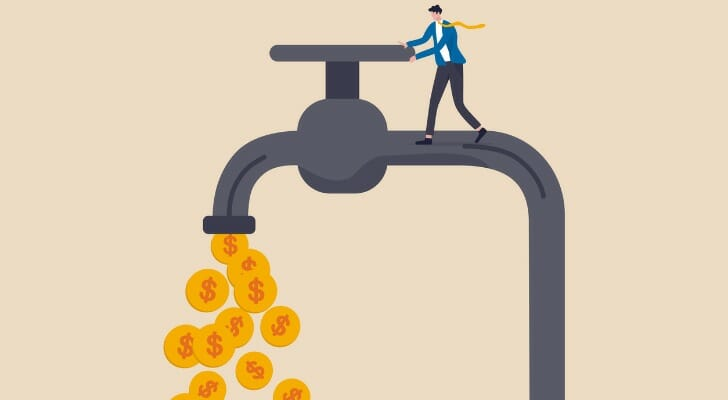# Dividend Rate vs. Dividend Yield: What They Tell Investors

ShareInvestors often put money into dividend-paying stocks for the income they generate. When it comes to finding dividend stocks there are numerous options out there. However, making the best financial decision relies on understanding this type of investment. To do that, you need to understand a dividend rate, sometimes just referred to as a dividend, and a dividend yield. Here are the differences investors should grasp regarding a dividend rate and a dividend yield. If you are thinking about including dividend-paying stocks in your investment strategy, consider speaking to a financial advisor.

## What Is a Dividend Rate?

dividend rate is the amount per share an investor receives at the time the dividend is paid out. It applies to a stock, as well as other investment vehicles like mutual funds and exchange-traded funds (ETFs).

So, if a stock pays an annual dividend at a semiannual rate, an investor receives two payments that total the full dividend amount. For example, the dividend rate can be an annual \$4 paid out two times per year at \$2 each of those two times. Most companies choose to pay at an annual, semiannual or monthly frequency, though.

A dividend rate is expressed using a dollar value. But it’s also important to note that some companies pay out these dividends in other forms, such as property or extra stock. They can also change their policy on the dividend rate.

## What Is a Dividend Yield?Dividend yield is a numerical figure describing the relationship between a stock’s annual dividend payment and its stock price. Dividend yield obviously changes as a stock price changes on the stock market, so know that when you use it you are only describing the dividend yield for the stock price at that moment. If the stock price changes drastically over the course of a market day, the dividend yield would change too.

Though dividends are often paid quarterly, for the purpose of dividend yield it is important to think about the dividend as an annual amount. Simply multiply the quarterly dividend by four to get the annual dividend, and use that figure when calculating the dividend yield for a given stock.

You can use multiple methods to find out whether a dividend yield is good or not. One way is to compare a company’s dividend yield to other sources of dividend yields, such as the S&P 500 and Treasurys.

## Dividend Rate vs. Dividend Yield: Example

To calculate a dividend rate, you must multiply the number of annual payment periods by the most recent dividend payment amount. Written as an equation, it looks like:

Latest Dividend Price x Dividend Frequency = Company Dividend Rate

As an example, consider a company called Company XYZ. The cash amount of its latest dividend was \$2.50 per share. It pays these dividends quarterly. Putting that into the equation, we see:

\$2.50 x 4 = \$10

So, the annual dividend rate for Company XYZ is \$10. If the company pays out any extra, non-recurring dividends, they simply add on to the total.

In contrast, finding the dividend yield requires you to divide the yearly dividend by the stock’s current price. Otherwise written out as:

Yearly Dividends Per Share / Share’s Current Price = Dividend Yield

We can add values to this formula to demonstrate. Let’s say that Company XYZ’s annual dividend payout per share is \$2.50. In addition, the company’s share price at the moment is \$240. If we put those numbers into the equation, we calculate:

\$2.50 / \$240 = 0.0104

Expressed as a percentage, that means Company XYZ’s dividend yield is 1.04%.

## The Takeaway

A dividend yield relies on multiple factors, including the dividend rate, which is simply the dollar amount a shareholder receives for each share owned. It’s more common to see a quoted dividend yield than a dividend rate. That’s because the former tells you the most efficient way to earn a return. If you’re considering fixed-income investing, take a look at Dividend Aristocrats. These are companies on the S&P 500 that have increased their dividends every year for the previous 25 years and met market capitalization and liquidity requirements.

## Tips on Investing

• While knowing the yield and rate of a stock’s dividend will help you form your financial decisions, you may not want to do it alone. A financial advisor has the professional experience on their side to assess whether a dividend’s yield suits your financial goals. You can receive the guidance of one easily with SmartAsset’s free matching tool. It pairs you with up in minutes with three local advisors for you to choose between. If you are ready to begin working towards your financial goals, start your search now.
• Dividend income is taxable. In particular, qualified dividends, which you will run into if you invest in U.S. based companies, are taxable at a long-term capital gains rate. Research which taxes and at what rate you may have to pay on your dividend-paying investments with SmartAsset’s free capital gains calculator.